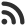Feed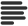Articles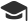Tutorials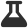Lab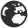CompaniesLeaderboard
 DG Student at BIT Mesra Updated Aug. 20, 2020, 10:02 a.m. ⋅ 953 views

# Goldman Sachs Placement papers Aptitude questions with answers

Goldman Sachs conducts an aptitude test and logical reasoning test during its off campus drive. Here are some aptitude questions you should practice upon, if you are planning to participate in Goldman sachs off campus drive.

You can find logical reasoning questions to practice from here : Goldman Sachs placement paper - Logical Reasoning Questions with answers

1. How many cubes one coloured silver on one face, orange or pink on another face and have four uncoloured faces ?

A. 8
B. 10
C. 12
D. 16

Ans. A

2. If a person walks at 14 km/hr instead of 10 km/hr, he would have walked 20 km more. The actual distance traveled by him is:

A. 70 km
B. 80 km
C. 50 km
D. 56 km

Ans. C

3. A set contains all numbers from 1 to 250. If a number is picked at random, what is the probability that it is a multiple of 3?

A. 82/250
B. 83/250
C. 80/250
D. 1/3

Ans. A

4. The speed of a train is 90 kmph. What is the distance covered by it in 10 minutes?

A. 15 kmph
B. 12 kmph
C. 10 kmph
D. 5 kmph

Ans. A

5. How many cubes have at least two faces coloured ?

A. 19
B. 20
C. 21
D. 23

Ans. C

6. Fill pipe A is 3 times faster than second Fill pipe B and takes 32 minutes less than Fill pipe B. When will the cistern be full if both pipes are opened together?

A. 25 minutes
B. 24 minutes
C. 30 minutes
D. 12 minutes

Ans. D

7. A sum was put at simple interest at a certain rate for 3 years. Had it been put at 1% higher rate, it would have fetched Rs. 5,100 more. The sum is :

A. Rs. 1,70,000
B. Rs. 1,50,000
C. Rs. 1,25,000
D. Rs. 1,20,000

Ans. A

8. A cistern can be filled by a tap in 3 hours while it can be emptied by another tap in 8 hours. If both the taps are opened simultaneously, then after how much time will the cistern get filled?

A. 4.8 hr
B. 2.4 hr
C. 3.6 hr
D. 1.8 hr

Ans. A

9. The tax on a commodity is diminished by 10 % and its consumption increased by 10 %. The effect on the revenue derived from it changes by K %. Find the value of K.

A. 1
B. 2
C. -1
D. -2

Ans. C

10. a, b, c and d are four numbers in arithmetic progression. The mean of these four numbers is 20. The common difference between the numbers is 5.
Find the product of first and last numbers.

A. 133.76
B. 343.75
C. 442.25
D. 124

Ans. B

11. What comes next in the series 8, 15, 12, 19, 16, 23?

A. 30
B. 20
C. 26
D. 31

Ans. B

10. A portion of a 30 m long tree is broken by a tornado and the top strikes the ground making an angle of 30° with the ground level. The height of the point where the tree is broken is equal to

A. 30/√3 m
B. 10 m
C. 30√3 m
D. 60 m

Ans. B

13. Two numbers are in the ratio xy,when 2 is added to both the numbers.the ratio becomes 1:2.when 3 is subtracted from both the numbers. The ratio becomes 1:3.Find the sum of x and y.

A. 27
B. 24
C. 28
D. 26

Ans. D

14. If 5 students utilize 18 pencils in 9 days, how long at the same rate will 66 pencils last for 15 students?

A. 10 days
B. 12 days
C. 11 days
D. None of these

Ans. C

15. The distance between two cities P and Q is 300km. A train starts from station P at 10 am with speed 80 km/hr towards Q. Another train starts from Q towards P with speed 40km/hr at 11 am. At what time do they meet.

A. 12:50 pm
B. 1 pm
C. 12:20 pm
D. 12:40 pm

Ans. A

16. A man rows 8 km/hr in still water. If the river is running at 2 km/hr, it takes 32 minutes to row to a place and back. How far is the place?

A. 1.5 km
B. 2.5 km
C. 2 km
D. 3 km

Ans. C

17. From a point A on the ground, the angle of elevation of a tower is 45˚. A ship moving at 20 m/sec started moving from point A to point B in 45 seconds. The angle of elevation from point B is 60˚. Find the height of the tower.

A. 1350 +450√3
B. 1350√3 + 450
C. 1800
D. None of the above

Ans. A

18. Anil is at present one-fourth the age of his father. After 16 years he will be one-half of the age of his father. Find the present age of Anil’s father.

A. 40 years
B. 36 years
C. 32 years
D. 28 years

Ans. C

19. A person on tour has Rs. 360 for his daily expenses. He decides to extend his tour program by 4 days which leads to cutting down daily expenses by Rs. 3 a day. The number of days of his tour program is

A. 15
B. 20
C. 18
D. 16

Ans. B

20. A man sitting in a train travelling at the rate of 50 km/hr observes that it takes 9 sec for a goods train travelling in the opposite direction to pass him. If the goods train is 187.5 m long, find its speed.

A. 25 km/hr
B. 40 km/hr
C. 35 km/hr
D. 36 km/hr

Ans. A

21. A shopkeeper has two items A & B. A was sold at a profit of 25% and B was sold at a loss of 15%. If the cost price of A is 15% more than that of B, what is the overall profit/ loss % to the shopkeeper?

A. 20.65
B. 19.65
C. 18.65
D. 20

Ans. B

22. Find the next number in the series :

8, 40, 840, ?

A. 326440
B. 324404
C. 353640
D. 352484

Ans. C

Events

Nov. 28, 2018, 5:30 p.m.

Python from zero to hero

place Delhi

Aug. 13, 2018, 5:30 p.m.

Python from zero to hero

place Bangalore ( HackersFriend office BTM Layout)